# Cross mapping

## API

New cross mapping API

In CausalityTools v1.6, the cross mapping API has been completely rewritten. The old syntax (both for convergent cross mapping and for pairwise asymmetric inference) will give a deprecation warning, but still works, and contains a bug fix. The old syntax will be abandoned in CausalityTools v2.0, so if writing new code, you should use the syntax below. You're now reading the documentation for the new API.

The cross mapping API consists of the following functions.

These functions can dispatch on a CrossmapMeasure, and we currently implement

CausalityTools.crossmapFunction
crossmap(measure::CrossmapMeasure, t̄::AbstractVector, S̄::AbstractDataset) → ρ
crossmap(measure::CrossmapMeasure, target::AbstractVector, source::AbstractVector) → ρ

Compute the cross map estimates between time-aligned time series t̄ and source embedding S̄, or between raw time series t and s.

This is just a wrapper around predict that simply returns the correspondence measure between the source and the target.

source
CausalityTools.predictFunction
predict(measure::CrossmapMeasure, t̄::AbstractVector, S̄::AbstractDataset) → t̂ₛ
predict(measure::CrossmapMeasure, target::AbstractVector, source::AbstractVector) → t̂ₛ, t̄, ρ

Perform point-wise cross mappings between source embeddings and target time series according to the algorithm specified by the given cross-map measure (e.g. ConvergentCrossMapping or PairwiseAsymmetricInference).

• First method: Returns a vector of predictions t̂ₛ (t̂ₛ := "predictions of t̄ based on source embedding S̄"), where t̂ₛ[i] is the prediction for t̄[i]. It assumes pre-embedded data which have been correctly time-aligned using a joint embedding (see embed), i.e. such that t̄[i] and S̄[i] correspond to the same time index.
• Second method: Jointly embeds the target and source time series (according to measure) to obtain time-index aligned target timeseries t̄ and source embedding S̄ (which is now a Dataset). Then calls predict(measure, t̄, S̄) (the first method), and returns both the predictions t̂ₛ, observations t̄ and their correspondence ρ according to measure.

Description

For each i ∈ {1, 2, …, N} where N = length(t) == length(s), we make the prediction t̂[i] (an estimate of t[i]) based on a linear combination of D + 1 other points in t, where the selection of points and weights for the linear combination are determined by the D+1 nearest neighbors of the point S̄[i]. The details of point selection and weights depend on measure.

Note: Some CrossmapMeasures may define more general mapping procedures. If so, the algorithm is described in their docstring.

source

## Measures

CausalityTools.ConvergentCrossMappingType
ConvergentCrossMapping <: CrossmapMeasure
ConvergentCrossMapping(; d::Int = 2, τ::Int = -1, w::Int = 0,
f = Statistics.cor, embed_warn = true)

The convergent cross mapping measure (Sugihara et al., 2012)[Sugihara2012]).

Specifies embedding dimension d, embedding lag τ to be used, as described below, with predict or crossmap. The Theiler window w controls how many temporal neighbors are excluded during neighbor searches (w = 0 means that only the point itself is excluded). f is a function that computes the agreement between observations and predictions (the default, f = Statistics.cor, gives the Pearson correlation coefficient).

Embedding

Let S(i) be the source time series variable and T(i) be the target time series variable. This version produces regular embeddings with fixed dimension d and embedding lag τ as follows:

$$$( S(i), S(i+\tau), S(i+2\tau), \ldots, S(i+(d-1)\tau, T(i))_{i=1}^{N-(d-1)\tau}.$$$

In this joint embedding, neighbor searches are performed in the subspace spanned by the first D-1 variables, while the last (D-th) variable is to be predicted.

With this convention, τ < 0 implies "past/present values of source used to predict target", and τ > 0 implies "future/present values of source used to predict target". The latter case may not be meaningful for many applications, so by default, a warning will be given if τ > 0 (embed_warn = false turns off warnings).

source
CausalityTools.PairwiseAsymmetricInferenceType
PairwiseAsymmetricInference <: CrossmapMeasure
PairwiseAsymmetricInference(; d::Int = 2, τ::Int = -1, w::Int = 0,
f = Statistics.cor, embed_warn = true)

The pairwise asymmetric inference (PAI) measure (McCracken & Weigel (2014)[McCracken2014]) is a version of ConvergentCrossMapping that searches for neighbors in mixed embeddings (i.e. both source and target variables included); otherwise, the algorithms are identical.

Specifies embedding dimension d, embedding lag τ to be used, as described below, with predict or crossmap. The Theiler window w controls how many temporal neighbors are excluded during neighbor searches (w = 0 means that only the point itself is excluded). f is a function that computes the agreement between observations and predictions (the default, f = Statistics.cor, gives the Pearson correlation coefficient).

Embedding

There are many possible ways of defining the embedding for PAI. Currently, we only implement the "add one non-lagged source timeseries to an embedding of the target" approach, which is used as an example in McCracken & Weigel's paper. Specifically: Let S(i) be the source time series variable and T(i) be the target time series variable. PairwiseAsymmetricInference produces regular embeddings with fixed dimension d and embedding lag τ as follows:

$$$(S(i), T(i+(d-1)\tau, \ldots, T(i+2\tau), T(i+\tau), T(i)))_{i=1}^{N-(d-1)\tau}.$$$

In this joint embedding, neighbor searches are performed in the subspace spanned by the first D variables, while the last variable is to be predicted.

With this convention, τ < 0 implies "past/present values of source used to predict target", and τ > 0 implies "future/present values of source used to predict target". The latter case may not be meaningful for many applications, so by default, a warning will be given if τ > 0 (embed_warn = false turns off warnings).

source

## Estimators

CausalityTools.CrossmapEstimatorType
CrossmapEstimator{LIBSIZES, RNG}

A parametric supertype for all cross-map estimators, which are used with predict and crossmap.

Because the type of the library may differ between estimators, and because RNGs from different packages may be used, subtypes must implement the LIBSIZES and RNG type parameters.

For efficiency purposes, subtypes may contain mutable containers that can be re-used for ensemble analysis (see Ensemble).

Libraries

A cross-map estimator uses the concept of "libraries". A library is essentially just a reference to a set of points, and usually, a library refers to indices of points, not the actual points themselves.

For example, for timeseries, RandomVectors(libsizes = 50:25:100) produces three separate libraries, where the first contains 50 randomly selected time indices, the second contains 75 randomly selected time indices, and the third contains 100 randomly selected time indices. This of course assumes that all quantities involved can be indexed using the same time indices, meaning that the concept of "library" only makes sense after relevant quantities have been jointly embedded, so that they can be jointly indexed. For non-instantaneous prediction, the maximum possible library size shrinks with the magnitude of the index/time-offset for the prediction.

For spatial analyses (not yet implemented), indices could be more complex and involve multi-indices.

source
CausalityTools.RandomVectorsType
RandomVectors <: CrossmapEstimator
RandomVectors(; libsizes, replace = false, rng = Random.default_rng())

Cross-map over N different libraries, where N = length(libsizes), and the i-th library has cardinality k = libsizes[i]. Points within each library are randomly selected, independently of other libraries, and replace controls whether or not to sample with replacement. A user-specified rng may be specified for reproducibility.

This is method 3 from Luo et al. (2015)[Luo2015].

See also: CrossmapEstimator.

source
CausalityTools.RandomSegmentType
RandomSegment <: CrossmapEstimator
RandomSegment(; libsizes::Int, rng = Random.default_rng())

Indicatates that cross mapping is performed on contiguous time series segments/windows of length L with a randomly selected starting point.

This is method 2 from Luo et al. (2015)[Luo2015].

source
CausalityTools.ExpandingSegmentType
ExpandingSegment <: CrossmapEstimator
ExpandingSegment(; libsizes::Int, rng = Random.default_rng())

Indicatates that cross mapping is performed on a contiguous time series segment/window, starting from the first available data point up to the Lth data point.

If used in an ensemble setting, the estimator is applied to time indices Lmin:step:Lmax of the joint embedding.

source

## Examples

### Convergent cross mapping (reproducing Sugihara et al., 2012)

Run blocks consecutively

If copying these examples and running them locally, make sure the relevant packages (given in the first block) are loaded first.

#### Figures 3C and 3D

Let's reproduce figures 3C and 3D in Sugihara et al. (2012)[Sugihara2012], which introduced the ConvergentCrossMapping measure. Equations and parameters can be found in their supplementary material. Simulatenously, we also compute the PairwiseAsymmetricInference measure from McCracken & Weigel (2014)[McCracken2014], which is a related method, but uses a slightly different embedding.

using CausalityTools
using Statistics
using LabelledArrays
using StaticArrays
using DynamicalSystemsBase
using StateSpaceSets
using CairoMakie, Printf

# -----------------------------------------------------------------------------------------
# Create 400-point long time series for Sugihara et al. (2012)'s example for figure 3.
# -----------------------------------------------------------------------------------------
function eom_logistic_sugi(u, p, t)
(; rx, ry, βxy, βyx) = p
(; x, y) = u

dx = x*(rx - rx*x - βxy*y)
dy = y*(ry - ry*y - βyx*x)
return SVector{2}(dx, dy)
end

# βxy := effect on x of y
# βyx := effect on y of x
function logistic_sugi(; u0 = rand(2), rx, ry, βxy, βyx)
p = @LArray [rx, ry, βxy, βyx] (:rx, :ry, :βxy, :βyx)
DiscreteDynamicalSystem(eom_logistic_sugi, u0, p)
end

sys_unidir = logistic_sugi(; u0 = [0.2, 0.4], rx = 3.7, ry = 3.700001, βxy = 0.00, βyx = 0.32);
x, y = columns(trajectory(sys_unidir, 1000, Ttr = 10000));

# -----------------------------------------------------------------------------------------
# Cross map.
# -----------------------------------------------------------------------------------------
m_ccm = ConvergentCrossMapping(d = 2)
m_pai = PairwiseAsymmetricInference(d = 2)
# Make predictions x̂y, i.e. predictions x̂ made from embedding of y (AND x, if PAI)
t̂ccm_x̂y, tccm_x̂y, ρccm_x̂y = predict(m_ccm, x, y)
t̂pai_x̂y, tpai_x̂y, ρpai_x̂y = predict(m_pai, x, y);
# Make predictions ŷx, i.e. predictions ŷ made from embedding of x (AND y, if PAI)
t̂ccm_ŷx, tccm_ŷx, ρccm_ŷx = predict(m_ccm, y, x)
t̂pai_ŷx, tpai_ŷx, ρpai_ŷx = predict(m_pai, y, x);

# -----------------------------------------------------------------------------------------
# Plot results
# -----------------------------------------------------------------------------------------
ρs = (ρccm_x̂y, ρpai_x̂y, ρccm_ŷx, ρpai_ŷx)
sccm_x̂y, spai_x̂y, sccm_ŷx, spai_ŷx = (map(ρ -> (@sprintf "%.3f" ρ), ρs)...,)

ρs = (ρccm_x̂y, ρpai_x̂y, ρccm_ŷx, ρpai_ŷx)
sccm_x̂y, spai_x̂y, sccm_ŷx, spai_ŷx = (map(ρ -> (@sprintf "%.3f" ρ), ρs)...,)

with_theme(theme_minimal(),
markersize = 5) do
fig = Figure();
ax_ŷx = Axis(fig[2,1], aspect = 1, xlabel = "y(t) (observed)", ylabel = "ŷ(t) | x (predicted)")
ax_x̂y = Axis(fig[2,2], aspect = 1, xlabel = "x(t) (observed)", ylabel = "x̂(t) | y (predicted)")
xlims!(ax_ŷx, (0, 1)), ylims!(ax_ŷx, (0, 1))
xlims!(ax_x̂y, (0, 1)), ylims!(ax_x̂y, (0, 1))
ax_ts = Axis(fig[1, 1:2], xlabel = "Time (t)", ylabel = "Value")
scatterlines!(ax_ts, x[1:300], label = "x")
scatterlines!(ax_ts, y[1:300], label = "y")
axislegend()
scatter!(ax_ŷx, tccm_ŷx, t̂ccm_ŷx, label = "CCM (ρ = $sccm_ŷx)", color = :black) scatter!(ax_ŷx, tpai_ŷx, t̂pai_ŷx, label = "PAI (ρ =$spai_ŷx)", color = :red)
axislegend(ax_ŷx, position = :lt)
scatter!(ax_x̂y, tccm_x̂y, t̂ccm_x̂y, label = "CCM (ρ = $sccm_x̂y)", color = :black) scatter!(ax_x̂y, tpai_x̂y, t̂pai_x̂y, label = "PAI (ρ =$spai_x̂y)", color = :red)
axislegend(ax_x̂y, position = :lt)
fig
end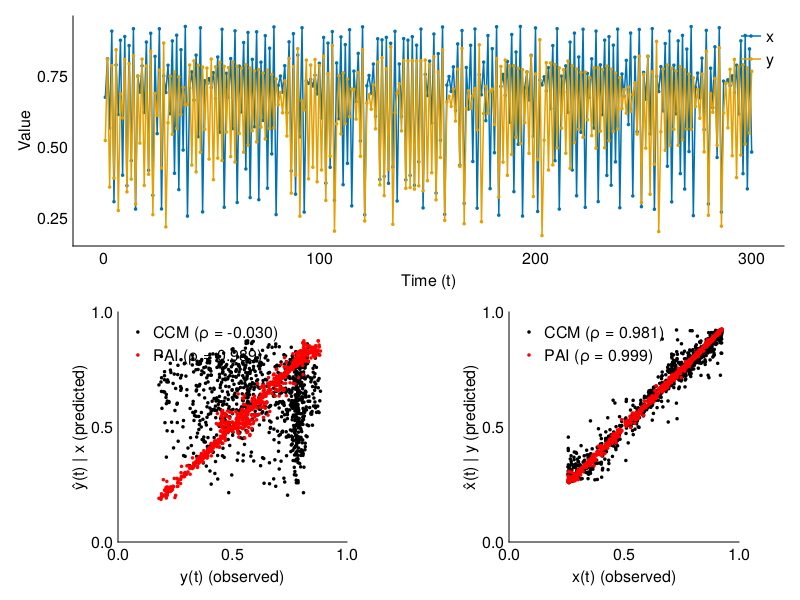#### Figure 3A

Let's reproduce figure 3A too, focusing only on ConvergentCrossMapping this time. In this figure, they compute the cross mapping for libraries of increasing size, always starting at time index 1. This approach - which we here call the ExpandingSegment estimator - is one of many ways of estimating the correspondence between observed and predicted value.

For this example, they use a bidirectional system with asymmetrical coupling strength.

using CausalityTools
using Statistics
using LabelledArrays
using StaticArrays
using DynamicalSystemsBase
using StateSpaceSets
using CairoMakie, Printf
# Used in reproduce_figure_3A_naive, and reproduce_figure_3A_ensemble below.
function add_to_fig!(fig_pos, libsizes, ρs_x̂y, ρs_ŷx; title = "", quantiles = false)
ax = Axis(fig_pos; title, aspect = 1,
xlabel = "Library size", ylabel = "Correlation (ρ)")
ylims!(ax, (-1, 1))
hlines!(, linestyle = :dash, alpha = 0.5, color = :grey)
scatterlines!(libsizes, median.(ρs_x̂y), label = "x̂|y", color = :blue)
scatterlines!(libsizes, median.(ρs_ŷx), label = "ŷ|x", color = :red)
if quantiles
band!(libsizes, quantile.(ρs_x̂y, 0.05), quantile.(ρs_x̂y, 0.95), color = (:blue, 0.5))
band!(libsizes, quantile.(ρs_ŷx, 0.05), quantile.(ρs_ŷx, 0.95), color = (:red, 0.5))
end
axislegend(ax, position = :rb)
end

function reproduce_figure_3A_naive(measure::CrossmapMeasure)
sys_bidir = logistic_sugi(; u0 = [0.2, 0.4], rx = 3.7, ry = 3.700001, βxy = 0.02, βyx = 0.32);
x, y = columns(trajectory(sys_bidir, 3100, Ttr = 10000));
libsizes = [20:2:50; 55:5:200; 300:50:500; 600:100:900; 1000:500:3000]
est = ExpandingSegment(; libsizes);
ρs_x̂y = crossmap(measure, est, x, y)
ρs_ŷx = crossmap(measure, est, y, x)

with_theme(theme_minimal(),
markersize = 5) do
fig = Figure(resolution = (800, 300))
add_to_fig!(fig[1, 1], libsizes, ρs_x̂y, ρs_ŷx; title = "ExpandingSegment")
fig
end
end

reproduce_figure_3A_naive(ConvergentCrossMapping(d = 3))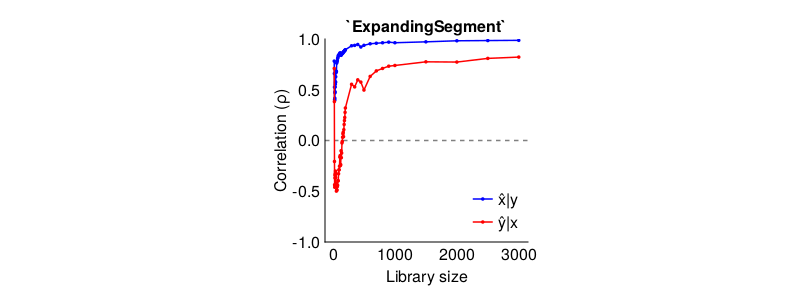Hm. This looks a bit like the paper, but the curve is not smooth. We can do better!

It is not clear from the paper exactly what they plot in their Figure 3A, if they plot an average of some kind, or precisely what parameters and initial conditions they use. However, we can get a smoother plot by using a Ensemble. Combined with a CrossmapEstimator, it uses Monte Carlo resampling on subsets of the input data to compute an ensemble of ρs that we here use to compute the median and 90-th percentile range for each library size.

function reproduce_figure_3A_ensemble(measure::CrossmapMeasure)
sys_bidir = logistic_sugi(; u0 = [0.4, 0.2], rx = 3.8, ry = 3.5, βxy = 0.02, βyx = 0.1);
x, y = columns(trajectory(sys_bidir, 10000, Ttr = 10000));
# Note: our time series are 1000 points long. When embedding, some points are
# lost, so we must use slightly less points for the segments than
# there are points in the original time series.
libsizes = [20:5:50; 55:5:200; 300:50:500; 600:100:900; 1000:500:3000]
# No point in doing more than one rep, because there data are always the same
# for ExpandingSegment.
ensemble_ev = Ensemble(measure, ExpandingSegment(; libsizes); nreps = 1)
ensemble_rs = Ensemble(measure, RandomSegment(; libsizes); nreps = 50)
ensemble_rv = Ensemble(measure, RandomVectors(; libsizes); nreps = 50)
ρs_x̂y_es = crossmap(ensemble_ev, x, y)
ρs_ŷx_es = crossmap(ensemble_ev, y, x)
ρs_x̂y_rs = crossmap(ensemble_rs, x, y)
ρs_ŷx_rs = crossmap(ensemble_rs, y, x)
ρs_x̂y_rv = crossmap(ensemble_rv, x, y)
ρs_ŷx_rv = crossmap(ensemble_rv, y, x)

with_theme(theme_minimal(),
markersize = 5) do
fig = Figure(resolution = (800, 300))
add_to_fig!(fig[1, 1], libsizes, ρs_x̂y_es, ρs_ŷx_es; title = "ExpandingSegment", quantiles = false) # quantiles make no sense for ExpandingSegment
add_to_fig!(fig[1, 2], libsizes, ρs_x̂y_rs, ρs_ŷx_rs; title = "RandomSegment", quantiles = true)
add_to_fig!(fig[1, 3], libsizes, ρs_x̂y_rv, ρs_ŷx_rv; title = "RandomVector", quantiles = true)
fig
end
end

reproduce_figure_3A_ensemble(ConvergentCrossMapping(d = 3, τ = -1))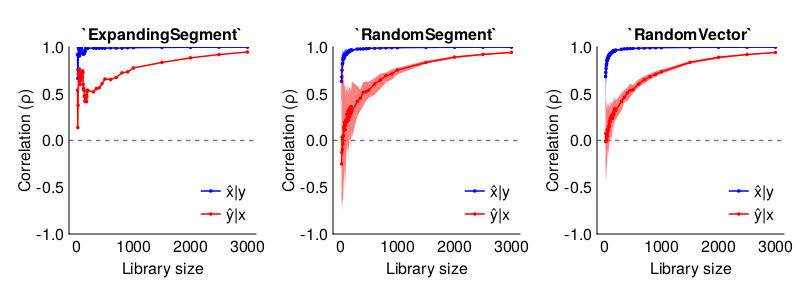With the RandomVector estimator, the mean of our ensemble ρs seem to look pretty much identical to Figure 3A in Sugihara et al. The RandomSegment estimator also performs pretty well, but since subsampled segments are contiguous, there are probably some autocorrelation effects at play.

We can avoid the autocorrelation issue by tuning the w parameter of the ConvergentCrossMapping measure, which is the Theiler window. Setting the Theiler window to w > 0, we can exclude neighbors of a query point p that are close to p in time, and thus deal with autocorrelation issues that way (the default w = 0 excludes only the point itself). Let's re-do the analysis with w = 5, just for fun.

reproduce_figure_3A_ensemble(ConvergentCrossMapping(d = 3, τ = -1, w = 5))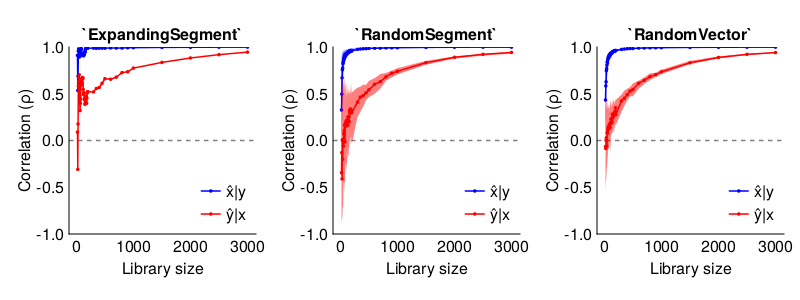There wasn't really that much of a difference, since for the logistic map, the autocorrelation function flips sign for every lag increase. However, for examples from other systems, tuning w may be important.

#### Figure 3B

What about figure 3B? Here they generate time series of length 400 for a range of values for both coupling parameters, and plot the dominant direction $\Delta = \rho(\hat{x} | y) - \rho(\hat{y} | x)$.

In the paper, they use a 1000 different parameterizations for the logistic map parameters, but don't state what is summarized in the plot. For simplicity, we'll therefore just stick to rx = ry = 3.7, as in the examples above, and just loop over the coupling strengths in either direction.

function reproduce_figure_3B()
βxys = 0.0:0.02:0.4
βyxs = 0.0:0.02:0.4
ρx̂ys = zeros(length(βxys), length(βyxs))
ρŷxs = zeros(length(βxys), length(βyxs))

for (i, βxy) in enumerate(βxys)
for (j, βyx) in enumerate(βyxs)
sys_bidir = logistic_sugi(; u0 = [0.2, 0.4], rx = 3.7, ry = 3.7, βxy, βyx);
# Generate 1000 points. Randomly select a 400-pt long segment.
x, y = columns(trajectory(sys_bidir, 1300, Ttr = 10000));
ensemble = Ensemble(CCM(d = 3, w = 5, τ = -1), RandomVectors(libsizes = 400), nreps = 10)
ρx̂ys[i, j] = mean(crossmap(ensemble, x, y))
ρŷxs[i, j] = mean(crossmap(ensemble, y, x))
end
end
Δ = ρŷxs .- ρx̂ys

with_theme(theme_minimal(),
markersize = 5) do
fig = Figure();
ax = Axis(fig[1, 1], xlabel = "βxy", ylabel = "βyx")
cont = contourf!(ax, Δ, levels = range(-1, 1, length = 10),
colormap = :curl)
ax.xticks = 1:length(βxys), string.([i % 2 == 0 ? βxys[i] : "" for i in 1:length(βxys)])
ax.yticks = 1:length(βyxs), string.([i % 2 == 0 ? βyxs[i] : "" for i in 1:length(βyxs)])
Colorbar(fig[1 ,2], cont, label = "Δ (ρ(ŷ|x) - ρ(x̂|y))")
tightlimits!(ax)
fig
end
end

reproduce_figure_3B()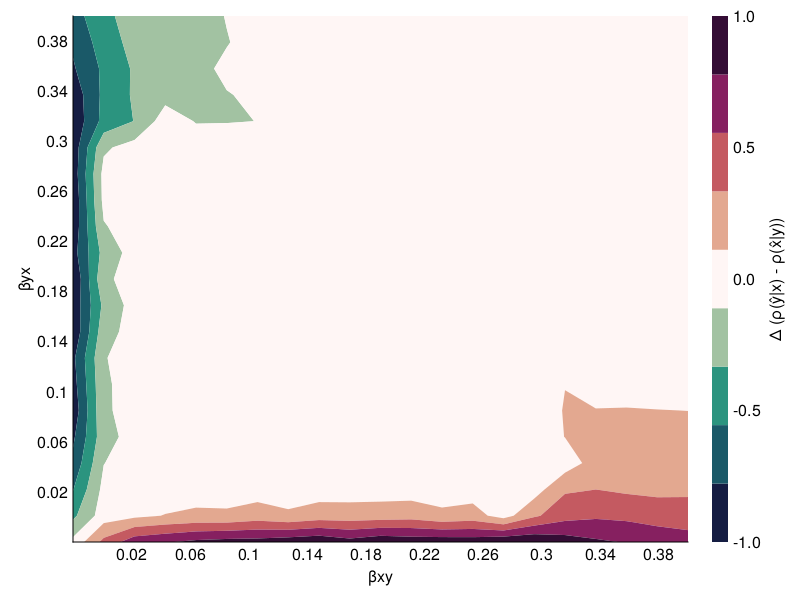### Pairwise asymmetric inference (reproducing McCracken & Weigel, 2014)

Let's try to reproduce figure 8 from McCracken & Weigel (2014)'s[McCracken2014] paper on PairwiseAsymmetricInference (PAI). We'll start by defining the their example B (equations 6-7). This system consists of two variables $X$ and $Y$, where $X$ drives $Y$.

After we have computed the PAI in both directions, we define a measure of directionality as the difference between PAI in the $X \to Y$ direction and in the $Y \to X$ direction, so that if $X$ drives $Y$, then $\Delta < 0$.

using CausalityTools
using LabelledArrays
using StaticArrays
using DynamicalSystemsBase
using StateSpaceSets
using CairoMakie, Printf
using Distributions: Normal
using Statistics: mean, std

function eom_nonlinear_sindriver(dx, x, p, n)
a, b, c, t, Δt = (p...,)
x, y = x, x
𝒩 = Normal(0, 1)

dx = sin(t)
dx = a*x * (1 - b*x) + c* rand(𝒩)
p[end-1] += 1 # update t

return
end

function nonlinear_sindriver(;u₀ = rand(2), a = 1.0, b = 1.0, c = 2.0, Δt = 1)
DiscreteDynamicalSystem(eom_nonlinear_sindriver, u₀, [a, b, c, 0, Δt])
end

function reproduce_figure_8_mccraken(;
c = 2.0, Δt = 0.2,
as = 0.25:0.25:5.0,
bs = 0.25:0.25:5.0)
# -----------------------------------------------------------------------------------------
# Generate many time series for many different values of the parameters a and b,
# and compute PAI. This will replicate the upper right panel of
# figure 8 in McCracken & Weigel (2014).
# -----------------------------------------------------------------------------------------

measure = PairwiseAsymmetricInference(d = 3)

# Manually resample nreps length-L time series and use mean ρ(x̂|X̄y) - ρ(ŷ|Ȳx)
# for each parameter combination.
nreps = 50
L = 300 # length of timeseries
Δ = zeros(length(as), length(bs))
for (i, a) in enumerate(as)
for (j, b) in enumerate(bs)
s = nonlinear_sindriver(; a, b, c,  Δt)
x, y = columns(trajectory(s, 1000, Ttr = 10000))
Δreps = zeros(nreps)
for i = 1:nreps
# Ensure we're subsampling at the same time indices.
ind_start = rand(1:(1000-L))
r = ind_start:(ind_start + L)
Δreps[i] = @views crossmap(measure, y[r], x[r]) -
crossmap(measure, x[r], y[r])
end
Δ[i, j] = mean(Δreps)
end
end

# -----------------------------------------------------------------------------------------
# An example time series for plotting.
# -----------------------------------------------------------------------------------------
sys = nonlinear_sindriver(; a = 1.0, b = 1.0, c, Δt)
npts = 500
orbit = trajectory(sys, npts, Ttr = 10000)
x, y = columns(orbit)
with_theme(theme_minimal(),
markersize = 5) do

X = x[1:300]
Y = y[1:300]
fig = Figure();
ax_ts = Axis(fig[1, 1:2], xlabel = "Time (t)", ylabel = "Value")
scatterlines!(ax_ts, (X .- mean(X)) ./ std(X), label = "x")
scatterlines!(ax_ts, (Y .- mean(Y)) ./ std(Y), label = "y")
axislegend()

ax_hm = Axis(fig[2, 1:2], xlabel = "a", ylabel = "b")
ax_hm.yticks = (1:length(as), string.([i % 2 == 0 ? as[i] : "" for i = 1:length(as)]))
ax_hm.xticks = (1:length(bs), string.([i % 2 == 0 ? bs[i] : "" for i = 1:length(bs)]))
hm = heatmap!(ax_hm, Δ,  colormap = :viridis)
Colorbar(fig[2, 3], hm; label = "Δ' = ρ(ŷ | yx) - ρ(x̂ | xy)")
fig
end
end

reproduce_figure_8_mccraken()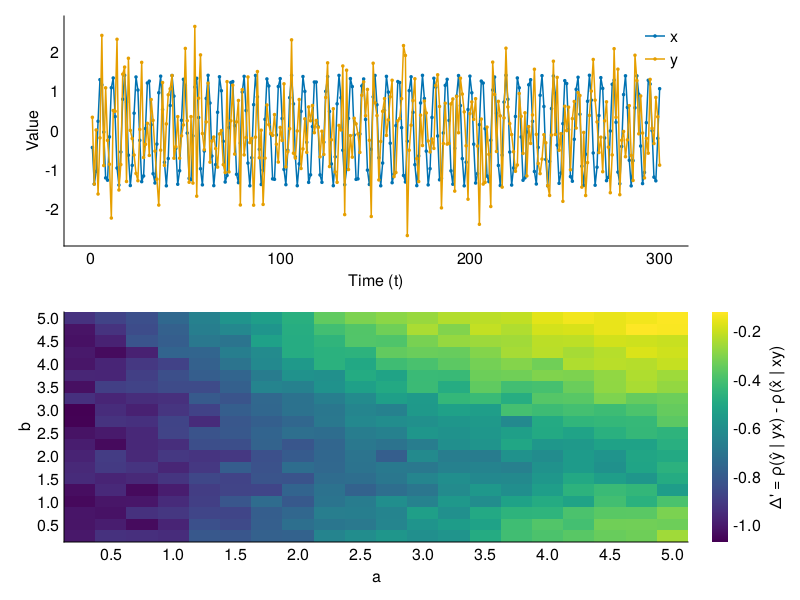As expected, $\Delta < 0$ for all parameter combinations, implying that $X$ "PAI drives" $Y$.

• Sugihara2012Sugihara, G., May, R., Ye, H., Hsieh, C. H., Deyle, E., Fogarty, M., & Munch, S. (2012). Detecting causality in complex ecosystems. science, 338(6106), 496-500.
• McCracken2014McCracken, J. M., & Weigel, R. S. (2014). Convergent cross-mapping and pairwise asymmetric inference. Physical Review E, 90(6), 062903.
• Luo2015"Questionable causality: Cosmic rays to temperature." Proceedings of the National Academy of Sciences Aug 2015, 112 (34) E4638-E4639; DOI: 10.1073/pnas.1510571112 Ming Luo, Holger Kantz, Ngar-Cheung Lau, Wenwen Huang, Yu Zhou.
• Luo2015"Questionable causality: Cosmic rays to temperature." Proceedings of the National Academy of Sciences Aug 2015, 112 (34) E4638-E4639; DOI: 10.1073/pnas.1510571112 Ming Luo, Holger Kantz, Ngar-Cheung Lau, Wenwen Huang, Yu Zhou.
• Sugihara2012Sugihara, G., May, R., Ye, H., Hsieh, C. H., Deyle, E., Fogarty, M., & Munch, S. (2012). Detecting causality in complex ecosystems. science, 338(6106), 496-500.
• McCracken2014McCracken, J. M., & Weigel, R. S. (2014). Convergent cross-mapping and pairwise asymmetric inference. Physical Review E, 90(6), 062903.